# Knapsack problem

•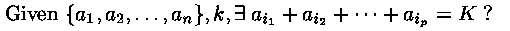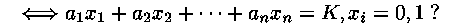General {an}: NP-complete, No polynomial time algorithm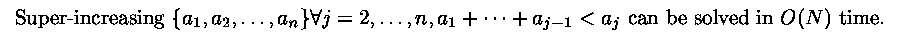Example: (3, 5, 9, 20, 44)
3x1+5x2+9x3+20x4+44x5=69 mod 89

3 < 5
3+5 < 9
3+5+9 < 20
3+5+9+20 < 44
```                           x5 = 1
=25       x4 = 1
=5              x3 = 0, x2 = 1
x1 = 0
```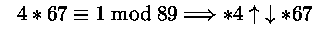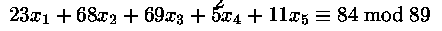• Knapsack Cipher System (Hellman & Merkle, 1978)
public key: (23, 68, 69, 5, 11), 89
private key: (3, 5, 9, 20, 44), 4

plaintext: M = (01011)2
ciphertext: M' = 84But Shamir (1983) showed, the method is breakable!